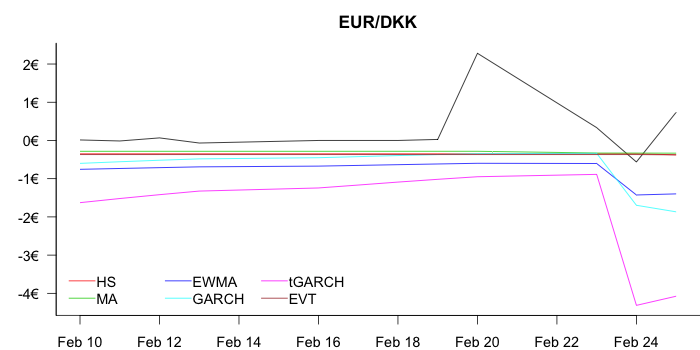The Danish FX event. Jon Danielsson. Modelsandrisk.org

### The Danish FX event

##### March 9, 2015
###### Denmark had a small FX event on March 20, in the context of the Swiss FX shock, it is not much a of an event, but it does reinforce stereotypes.

Denmark had a small FX event on March 20, in the context of the Swiss FX shock, it is not much a of an event, but it does reinforce stereotypes.

This happened because the Danish pension funds got worried that The Danish National Bank would follow its Swiss counterpart. They wanted to be long krona, so the exchange rate wobbled.

#### Starting with the Kronas to EUR#### and then EUR to Kronas#### The volatility measures are of course symmetric.

Why? Consider the equations

GARCH $$\sigma ^2_{t} =\omega +\alpha Y_{t-1}^{2}+\beta \sigma ^2_{t-1}$$ or $$y_t = \sigma_{t} Z_t$$ with $$Z_t\sim N(0,1)$$ or EWMA $$\sigma_t^2 = (1-\lambda) y_{t-1}^2 + \lambda \sigma_{t-1}^2.$$ tGARCH, same as GARCH, except $$Z_{t}\sim t_{\left( \nu \right) }.$$ and all of these react the same way regardless of whether $y_{t-1}<0$ or $y_{t-1}>0$.

So, if you don't like the symmetry, can try APARCH or something like that, and then accept the need for a much larger sample size. The 1000 used here is not enough.

and neither HS nor EVT react as they are in the former case completely insensitive to the tail event and the latter not very much.

Post-Crisis banking regulation: Evolution of economic thinking as it happened on Vox
Why risk is hard to measure

##### Models and risk
Bloggs and appendices on risk, models, regulations, cryptocurrencies and related topics